## 2.1 The Limit of a Function and Limit Laws

### Learning Objectives

• Using correct notation, describe the limit of a function.
• Use a table of values to estimate the limit of a function or to identify when the limit does not exist.
• Use a graph to estimate the limit of a function or to identify when the limit does not exist.
• Recognize the basic limit laws.
• Use the limit laws to evaluate the limit of a function.
• Evaluate the limit of a function by factoring.
• Use the limit laws to evaluate the limit of a polynomial or rational function.
• Evaluate the limit of a function by factoring or by using conjugates.
• Evaluate the limit of a function by using the squeeze theorem.

The concept of a limit or limiting process, essential to the understanding of calculus, has been around for thousands of years. In fact, early mathematicians used a limiting process to obtain better and better approximations of areas of circles. Yet, the formal definition of a limit—as we know and understand it today—did not appear until the late 19th century. We therefore begin our quest to understand limits, as our mathematical ancestors did, by using an intuitive approach. At the end of this chapter, armed with a conceptual understanding of limits, we examine the formal definition of a limit.

We begin our exploration of limits by taking a look at the graphs of the functions

$f(x)=\frac{x^2-4}{x-2}, \, g(x)=\frac{|x-2|}{x-2}$, and $h(x)=\frac{1}{(x-2)^2}$,

which are shown in (Figure). In particular, let’s focus our attention on the behavior of each graph at and around $x=2$.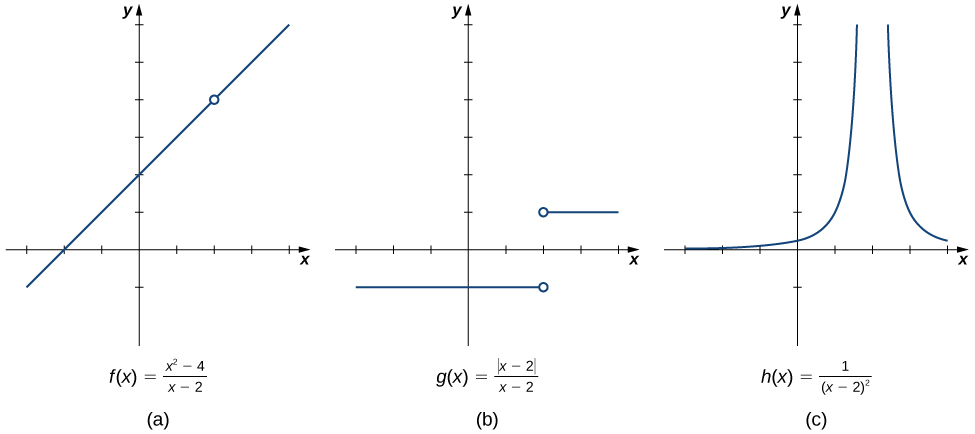Figure 1. These graphs show the behavior of three different functions around $x=2$.

Each of the three functions is undefined at $x=2$, but if we make this statement and no other, we give a very incomplete picture of how each function behaves in the vicinity of $x=2$. To express the behavior of each graph in the vicinity of 2 more completely, we need to introduce the concept of a limit.

# Intuitive Definition of a Limit

Let’s first take a closer look at how the function $f(x)=(x^2-4)/(x-2)$ behaves around $x=2$ in (Figure). As the values of $x$ approach 2 from either side of 2, the values of $y=f(x)$ approach 4. Mathematically, we say that the limit of $f(x)$ as $x$ approaches 2 is 4. Symbolically, we express this limit as

$\underset{x \to 2}{\lim}f(x)=4$.

From this very brief informal look at one limit, let’s start to develop an intuitive definition of the limit. We can think of the limit of a function at a number $a$ as being the one real number $L$ that the functional values approach as the $x$-values approach $a$, provided such a real number $L$ exists. Stated more carefully, we have the following definition:

### Definition

Let $f(x)$ be a function defined at all values in an open interval containing $a$, with the possible exception of $a$ itself, and let $L$ be a real number. If all values of the function $f(x)$ approach the real number $L$ as the values of $x(\ne a)$ approach the number $a$, then we say that the limit of $f(x)$ as $x$ approaches $a$ is $L$. (More succinct, as $x$ gets closer to $a$, $f(x)$ gets closer and stays close to $L$.) Symbolically, we express this idea as

$\underset{x\to a}{\lim}f(x)=L$.

We can estimate limits by constructing tables of functional values and by looking at their graphs. This process is described in the following Problem-Solving Strategy.

### Problem-Solving Strategy: Evaluating a Limit Using a Table of Functional Values

1. To evaluate $\underset{x\to a}{\lim}f(x)$, we begin by completing a table of functional values. We should choose two sets of $x$-values—one set of values approaching $a$ and less than $a$, and another set of values approaching $a$ and greater than $a$. (Figure) demonstrates what your tables might look like.
Table of Functional Values for $\underset{x\to a}{\lim}f(x)$
$x$ $f(x)$ $x$ $f(x)$
$a-0.1$ $f(a-0.1)$ $a+0.1$ $f(a+0.1)$
$a-0.01$ $f(a-0.01)$ $a+0.01$ $f(a+0.01)$
$a-0.001$ $f(a-0.001)$ $a+0.001$ $f(a+0.001)$
$a-0.0001$ $f(a-0.0001)$ $a+0.0001$ $f(a+0.0001)$
Use additional values as necessary. Use additional values as necessary.
2. Next, let’s look at the values in each of the $f(x)$ columns and determine whether the values seem to be approaching a single value as we move down each column. In our columns, we look at the sequence $f(a-0.1), \, f(a-0.01), \, f(a-0.001), \, f(a-0.0001),$ and so on, and $f(a+0.1), \, f(a+0.01), \, f(a+0.001), \, f(a+0.0001)$ and so on. (Note: Although we have chosen the $x$-values $a \pm 0.1, \, a \pm 0.01, \, a \pm 0.001, \, a \pm 0.0001$, and so forth, and these values will probably work nearly every time, on very rare occasions we may need to modify our choices.)
3. If both columns approach a common $y$-value $L$, we state $\underset{x\to a}{\lim}f(x)=L$. We can use the following strategy to confirm the result obtained from the table or as an alternative method for estimating a limit.
4. Using a graphing calculator or computer software that allows us graph functions, we can plot the function $f(x)$, making sure the functional values of $f(x)$ for $x$-values near $a$ are in our window. We can use the trace feature to move along the graph of the function and watch the $y$-value readout as the $x$-values approach $a$. If the $y$-values approach $L$ as our $x$-values approach $a$ from both directions, then $\underset{x\to a}{\lim}f(x)=L$. We may need to zoom in on our graph and repeat this process several times.

We apply this Problem-Solving Strategy to compute a limit in (Figure).

### Evaluating a Limit Using a Table of Functional Values 1

Evaluate $\underset{x\to 0}{\lim}\frac{\sin x}{x}$ using a table of functional values.

### Evaluating a Limit Using a Table of Functional Values 2

Evaluate $\underset{x\to 4}{\lim}\frac{\sqrt{x}-2}{x-4}$ using a table of functional values.

Estimate $\underset{x\to 1}{\lim}\frac{\frac{1}{x}-1}{x-1}$ using a table of functional values. Use a graph to confirm your estimate.

#### Hint

Use 0.9, 0.99, 0.999, 0.9999, 0.99999 and 1.1, 1.01, 1.001, 1.0001, 1.00001 as your table values.

At this point, we see from (Figure) and (Figure) that it may be just as easy, if not easier, to estimate a limit of a function by inspecting its graph as it is to estimate the limit by using a table of functional values. In (Figure), we evaluate a limit exclusively by looking at a graph rather than by using a table of functional values.

### Evaluating a Limit Using a Graph

For $g(x)$ shown in (Figure), evaluate $\underset{x\to -1}{\lim}g(x)$.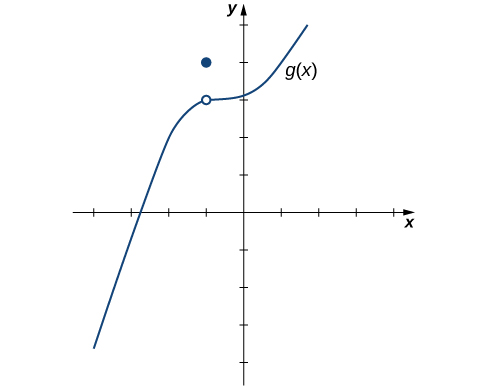Figure 4. The graph of $g(x)$ includes one value not on a smooth curve.

Based on (Figure), we make the following observation: It is possible for the limit of a function to exist at a point, and for the function to be defined at this point, but the limit of the function and the value of the function at the point may be different.

Use the graph of $h(x)$ in (Figure) to evaluate $\underset{x\to 2}{\lim}h(x)$, if possible.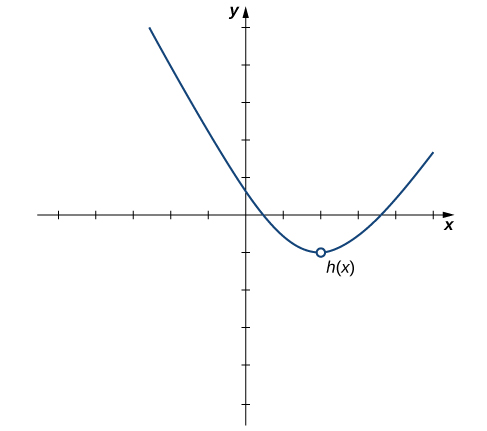Figure 5.  The graph of $h(x)$ consists of a smooth graph with a single removed point at $x=2$.

#### Hint

What $y$-value does the function approach as the $x$-values approach 2?

Looking at a table of functional values or looking at the graph of a function provides us with useful insight into the value of the limit of a function at a given point. However, these techniques rely too much on guesswork. We eventually need to develop alternative methods of evaluating limits. These new methods are more algebraic in nature and we explore them in the next section; however, at this point we introduce two special limits that are foundational to the techniques to come.

### Two Important Limits

Let $a$ be a real number and $c$ be a constant.

1. $\underset{x\to a}{\lim}x=a$
2. $\underset{x\to a}{\lim}c=c$

We can make the following observations about these two limits.

1. For the first limit, observe that as $x$ approaches $a$, so does $f(x)$, because $f(x)=x$. Consequently, $\underset{x\to a}{\lim}x=a$.
2. For the second limit, consider (Figure).
Table of Functional Values for $\underset{x\to a}{\lim}c=c$
$x$ $f(x)=c$ $x$ $f(x)=c$
$a-0.1$ $c$ $a+0.1$ $c$
$a-0.01$ $c$ $a+0.01$ $c$
$a-0.001$ $c$ $a+0.001$ $c$
$a-0.0001$ $c$ $a+0.0001$ $c$

Observe that for all values of $x$ (regardless of whether they are approaching $a$), the values $f(x)$ remain constant at $c$. We have no choice but to conclude $\underset{x\to a}{\lim}c=c$.

# The Existence of a Limit

As we consider the limit in the next example, keep in mind that for the limit of a function to exist at a point, the functional values must approach a single real-number value at that point. If the functional values do not approach a single value, then the limit does not exist.

### Evaluating a Limit That Fails to Exist

Evaluate $\underset{x\to 0}{\lim} \sin (1/x)$ using a table of values.

Use a table of functional values to evaluate $\underset{x\to 2}{\lim}\frac{|x^2-4|}{x-2}$, if possible.

#### Hint

Use $x$-values 1.9, 1.99, 1.999, 1.9999, 1.9999 and 2.1, 2.01, 2.001, 2.0001, 2.00001 in your table.

In the beginning of this section, we evaluated limits by looking at graphs or by constructing a table of values. Now we establish laws for calculating limits and learn how to apply these laws. In the Student Project at the end of this section, you have the opportunity to apply these limit laws to derive the formula for the area of a circle by adapting a method devised by the Greek mathematician Archimedes. We begin by restating two useful limit results from the previous section. These two results, together with the limit laws, serve as a foundation for calculating many limits.

# Evaluating Limits with the Limit Laws

The first two limit laws were stated in (Figure) and we repeat them here. These basic results, together with the other limit laws, allow us to evaluate limits of many algebraic functions.

### Basic Limit Results

For any real number $a$ and any constant $c$,

1. $\underset{x\to a}{\lim}x=a$
2. $\underset{x\to a}{\lim}c=c$

### Evaluating a Basic Limit

Evaluate each of the following limits using (Figure).

1. $\underset{x\to 2}{\lim}x$
2. $\underset{x\to 2}{\lim}5$

We now take a look at the limit laws, the individual properties of limits. The proofs that these laws hold are omitted here.

### Limit Laws

Let $f(x)$ and $g(x)$ be defined for all $x\ne a$ over some open interval containing $a$. Assume that $L$ and $M$ are real numbers such that $\underset{x\to a}{\lim}f(x)=L$ and $\underset{x\to a}{\lim}g(x)=M$. Let $c$ be a constant. Then, each of the following statements holds:

Sum law for limits: $\underset{x\to a}{\lim}(f(x)+g(x))=\underset{x\to a}{\lim}f(x)+\underset{x\to a}{\lim}g(x)=L+M$

Difference law for limits: $\underset{x\to a}{\lim}(f(x)-g(x))=\underset{x\to a}{\lim}f(x)-\underset{x\to a}{\lim}g(x)=L-M$

Constant multiple law for limits: $\underset{x\to a}{\lim}cf(x)=c \cdot \underset{x\to a}{\lim}f(x)=cL$

Product law for limits: $\underset{x\to a}{\lim}(f(x) \cdot g(x))=\underset{x\to a}{\lim}f(x) \cdot \underset{x\to a}{\lim}g(x)=L \cdot M$

Quotient law for limits: $\underset{x\to a}{\lim}\frac{f(x)}{g(x)}=\frac{\underset{x\to a}{\lim}f(x)}{\underset{x\to a}{\lim}g(x)}=\frac{L}{M}$ for $M\ne 0$

Power law for limits: $\underset{x\to a}{\lim}(f(x))^n=(\underset{x\to a}{\lim}f(x))^n=L^n$ for every positive integer $n$.

Root law for limits: $\underset{x\to a}{\lim}\sqrt[n]{f(x)}=\sqrt[n]{\underset{x\to a}{\lim}f(x)}=\sqrt[n]{L}$ for all $L$ if $n$ is odd and for $L\ge 0$ if $n$ is even.

We now practice applying these limit laws to evaluate a limit.

### Evaluating a Limit Using Limit Laws

Use the limit laws to evaluate $\underset{x\to -3}{\lim}(4x+2)$.

### Using Limit Laws Repeatedly

Use the limit laws to evaluate $\underset{x\to 2}{\lim}\frac{2x^2-3x+1}{x^3+4}$.

Use the limit laws to evaluate $\underset{x\to 6}{\lim}(2x-1)\sqrt{x+4}$. In each step, indicate the limit law applied.

#### Hint

Begin by applying the product law.

# Limits of Polynomial and Rational Functions

By now you have probably noticed that, in each of the previous examples, it has been the case that $\underset{x\to a}{\lim}f(x)=f(a)$. This is not always true, but it does hold for all polynomials for any choice of $a$ and for all rational functions at all values of $a$ for which the rational function is defined.

### Limits of Polynomial and Rational Functions

Let $p(x)$ and $q(x)$ be polynomial functions. Let $a$ be a real number. Then,

$\underset{x\to a}{\lim}p(x)=p(a)$
$\underset{x\to a}{\lim}\frac{p(x)}{q(x)}=\frac{p(a)}{q(a)} \, \text{when} \, q(a)\ne 0$.

To see that this theorem holds, consider the polynomial $p(x)=c_nx^n+c_{n-1}x^{n-1}+\cdots +c_1x+c_0$. By applying the sum, constant multiple, and power laws, we end up with

$\begin{array}{cc}\hfill \underset{x\to a}{\lim}p(x)& =\underset{x\to a}{\lim}(c_nx^n+c_{n-1}x^{n-1}+\cdots +c_1x+c_0)\hfill \\ & =c_n(\underset{x\to a}{\lim}x)^n+c_{n-1}(\underset{x\to a}{\lim}x)^{n-1}+\cdots +c_1(\underset{x\to a}{\lim}x)+\underset{x\to a}{\lim}c_0\hfill \\ & =c_na^n+c_{n-1}a^{n-1}+\cdots +c_1a+c_0\hfill \\ & =p(a)\hfill \end{array}$

It now follows from the quotient law that if $p(x)$ and $q(x)$ are polynomials for which $q(a)\ne 0$, then

$\underset{x\to a}{\lim}\frac{p(x)}{q(x)}=\frac{p(a)}{q(a)}$.

(Figure) applies this result.

### Evaluating a Limit of a Rational Function

Evaluate the $\underset{x\to 3}{\lim}\frac{2x^2-3x+1}{5x+4}$.

Evaluate $\underset{x\to -2}{\lim}(3x^3-2x+7)$.

Use (Figure)

# Additional Limit Evaluation Techniques

As we have seen, we may evaluate easily the limits of polynomials and limits of some (but not all) rational functions by direct substitution. However, as we saw in the introductory section on limits, it is certainly possible for $\underset{x\to a}{\lim}f(x)$ to exist when $f(a)$ is undefined. The following observation allows us to evaluate many limits of this type:

If for all $x\ne a, \, f(x)=g(x)$ over some open interval containing $a$, then $\underset{x\to a}{\lim}f(x)=\underset{x\to a}{\lim}g(x)$.

To understand this idea better, consider the limit $\underset{x\to 1}{\lim}\frac{x^2-1}{x-1}$.

The function

$\begin{array}{cc}\hfill f(x)& =\frac{x^2-1}{x-1}\hfill \\ & =\frac{(x-1)(x+1)}{x-1}\hfill \end{array}$

and the function $g(x)=x+1$ are identical for all values of $x\ne 1.$ The graphs of these two functions are shown in (Figure).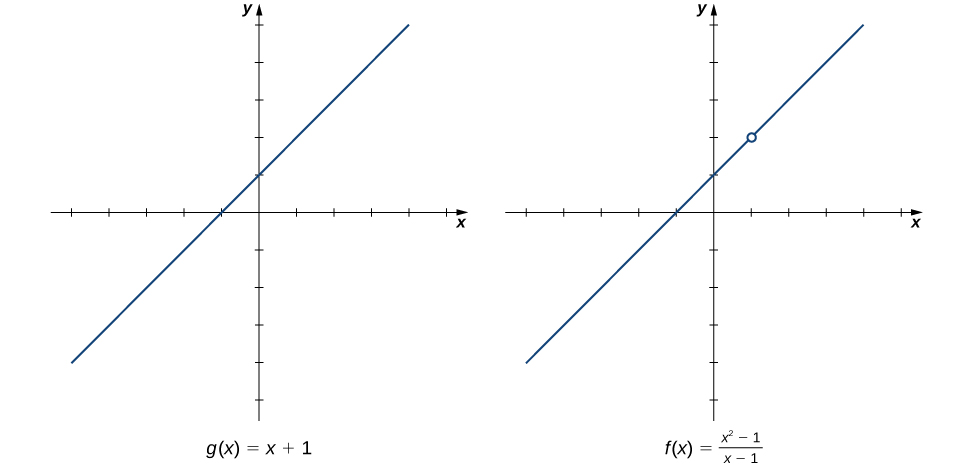Figure 1. The graphs of $f(x)$ and $g(x)$ are identical for all $x\ne 1$. Their limits at 1 are equal.

We see that

$\begin{array}{cc}\hfill \underset{x\to 1}{\lim}\frac{x^2-1}{x-1}& =\underset{x\to 1}{\lim}\frac{(x-1)(x+1)}{x-1}\hfill \\ & =\underset{x\to 1}{\lim}(x+1)\hfill \\ & =2\hfill \end{array}$

The limit has the form $\underset{x\to a}{\lim}\frac{f(x)}{g(x)}$, where $\underset{x\to a}{\lim}f(x)=0$ and $\underset{x\to a}{\lim}g(x)=0$. (In this case, we say that $f(x)/g(x)$ has the indeterminate form 0/0.) The following Problem-Solving Strategy provides a general outline for evaluating limits of this type.

### Problem-Solving Strategy: Calculating a Limit When $f(x)/g(x)$ has the Indeterminate Form 0/0

1. First, we need to make sure that our function has the appropriate form and cannot be evaluated immediately using the limit laws.
2. We then need to find a function that is equal to $h(x)=f(x)/g(x)$ for all $x\ne a$ over some interval containing $a$. To do this, we may need to try one or more of the following steps:
1. If $f(x)$ and $g(x)$ are polynomials, we should factor each function and cancel out any common factors.
2. If the numerator or denominator contains a difference involving a square root, we should try multiplying the numerator and denominator by the conjugate of the expression involving the square root.
3. If $f(x)/g(x)$ is a complex fraction, we begin by simplifying it.
3. Last, we apply the limit laws.

The next examples demonstrate the use of this Problem-Solving Strategy. (Figure) illustrates the factor-and-cancel technique; (Figure) shows multiplying by a conjugate. In (Figure), we look at simplifying a complex fraction.

### Evaluating a Limit by Factoring and Canceling

Evaluate $\underset{x\to 3}{\lim}\frac{x^2-3x}{2x^2-5x-3}$.

Evaluate $\underset{x\to -3}{\lim}\frac{x^2+4x+3}{x^2-9}$.

#### Hint

Follow the steps in the Problem-Solving Strategy and (Figure).

### Evaluating a Limit by Multiplying by a Conjugate

Evaluate $\underset{x\to -1}{\lim}\frac{\sqrt{x+2}-1}{x+1}$.

Evaluate $\underset{x\to 5}{\lim}\frac{\sqrt{x-1}-2}{x-5}$.

#### Hint

Follow the steps in the Problem-Solving Strategy and (Figure).

### Evaluating a Limit by Simplifying a Complex Fraction

Evaluate $\underset{x\to 1}{\lim}\frac{\frac{1}{x+1}-\frac{1}{2}}{x-1}$.

Evaluate $\underset{x\to -3}{\lim}\frac{\frac{1}{x+2}+1}{x+3}$.

#### Hint

Follow the steps in the Problem-Solving Strategy and (Figure).

(Figure) does not fall neatly into any of the patterns established in the previous examples. However, with a little creativity, we can still use these same techniques.

### Evaluating a Limit When the Limit Laws Do Not Apply

Evaluate $\underset{x\to 0}{\lim}\big(\frac{1}{x}+\frac{5}{x(x-5)}\big)$.

Evaluate $\underset{x\to 3}{\lim}(\frac{1}{x-3}-\frac{4}{x^2-2x-3})$.

#### Hint

Use the same technique as (Figure). Don’t forget to factor $x^2-2x-3$ before getting a common denominator.

# The Squeeze Theorem

The techniques we have developed thus far work very well for algebraic functions, but we are still unable to evaluate limits of very basic trigonometric functions. The next theorem, called the squeeze theorem, proves very useful for establishing basic trigonometric limits. This theorem allows us to calculate limits by “squeezing” a function, with a limit at a point $a$ that is unknown, between two functions having a common known limit at $a$. (Figure) illustrates this idea.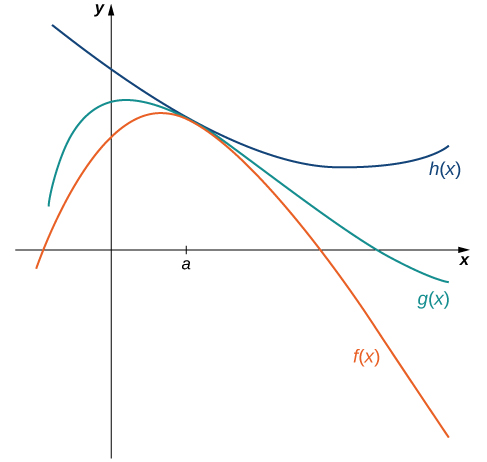Figure 4. The Squeeze Theorem applies when $f(x)\le g(x)\le h(x)$ and $\underset{x\to a}{\lim}f(x)=\underset{x\to a}{\lim}h(x)$.

### The Squeeze Theorem

Let $f(x), \, g(x)$, and $h(x)$ be defined for all $x\ne a$ over an open interval containing $a$. If

$f(x)\le g(x)\le h(x)$

for all $x\ne a$ in an open interval containing $a$ and

$\underset{x\to a}{\lim}f(x)=L=\underset{x\to a}{\lim}h(x)$

where $L$ is a real number, then $\underset{x\to a}{\lim}g(x)=L$.

### Applying the Squeeze Theorem

Apply the Squeeze Theorem to evaluate $\underset{x\to 0}{\lim}x \cos x$.

Use the Squeeze Theorem to evaluate $\underset{x\to 0}{\lim}x^2 \sin \frac{1}{x}$.

#### Hint

Use the fact that $-x^2\le x^2 \sin (1/x)\le x^2$ to help you find two functions such that $x^2 \sin (1/x)$ is squeezed between them.

### Deriving the Formula for the Area of a Circle

Some of the geometric formulas we take for granted today were first derived by methods that anticipate some of the methods of calculus. The Greek mathematician Archimedes (ca. 287−212 BCE) was particularly inventive, using polygons inscribed within circles to approximate the area of the circle as the number of sides of the polygon increased. He never came up with the idea of a limit, but we can use this idea to see what his geometric constructions could have predicted about the limit.

We can estimate the area of a circle by computing the area of an inscribed regular polygon. Think of the regular polygon as being made up of $n$ triangles. By taking the limit as the vertex angle of these triangles goes to zero, you can obtain the area of the circle. To see this, carry out the following steps:

1. Express the height $h$ and the base $b$ of the isosceles triangle in (Figure) in terms of $\theta$ and $r$.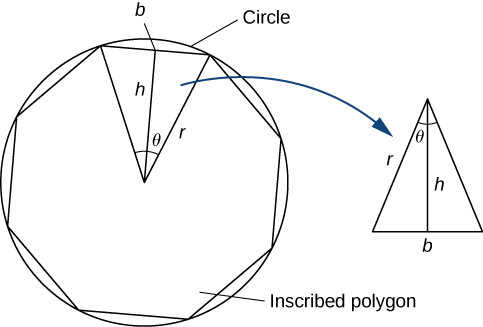Figure 8.

2. Using the expressions that you obtained in step 1, express the area of the isosceles triangle in terms of $\theta$ and $r$.
(Substitute $(1/2)\sin \theta$ for $\sin(\theta/2) \cos(\theta/2)$ in your expression.)
3. If an $n$-sided regular polygon is inscribed in a circle of radius $r$, find a relationship between $\theta$ and $n$. Solve this for $n$. Keep in mind there are $2\pi$ radians in a circle. (Use radians, not degrees.)
4. Find an expression for the area of the $n$-sided polygon in terms of $r$ and $\theta$.
5. To find a formula for the area of the circle, find the limit of the expression in step 4 as $\theta$ goes to zero. (Hint: $\underset{\theta \to 0}{\lim}\frac{(\sin \theta)}{\theta}=1$.)

The technique of estimating areas of regions by using polygons is revisited in Introduction to Integration.

### Key Concepts

• A table of values or graph may be used to estimate a limit.
• If the limit of a function at a point does not exist, it is still possible that the limits from the left and right at that point may exist.
• If the limits of a function from the left and right exist and are equal, then the limit of the function is that common value.
• We may use limits to describe infinite behavior of a function at a point.
• The limit laws allow us to evaluate limits of functions without having to go through step-by-step processes each time.
• For polynomials and rational functions, $\underset{x\to a}{\lim}f(x)=f(a)$.
• You can evaluate the limit of a function by factoring and canceling, by multiplying by a conjugate, or by simplifying a complex fraction.
• The Squeeze Theorem allows you to find the limit of a function if the function is always greater than one function and less than another function with limits that are known.

# Key Equations

• Intuitive Definition of the Limit
$\underset{x\to a}{\lim}f(x)=L$
• Basic Limit Results
$\underset{x\to a}{\lim}x=a$
$\underset{x\to a}{\lim}c=c$
• Important Limits
$\underset{\theta \to 0}{\lim} \sin \theta =0$
$\underset{\theta \to 0}{\lim} \cos \theta =1$

For the following exercises, consider the function $f(x)=\frac{x^2-1}{|x-1|}$.

1. [T] Complete the following table for the function. Round your solutions to four decimal places.

$x$ $f(x)$ $x$ $f(x)$
0.9 a. 1.1 e.
0.99 b. 1.01 f.
0.999 c. 1.001 g.
0.9999 d. 1.0001 h.

2. What do your results in the preceding exercise indicate about the two-sided limit $\underset{x\to 1}{\lim}f(x)$? Explain your response.

For the following exercises, consider the function $f(x)=(1+x)^{1/x}$.

3. [T] Make a table showing the values of $f$ for $x=-0.01, \, -0.001, \, -0.0001, \, -0.00001$ and for $x=0.01, \, 0.001, \, 0.0001, \, 0.00001$. Round your solutions to five decimal places.

$x$ $f(x)$ $x$ $f(x)$
−0.01 a. 0.01 e.
−0.001 b. 0.001 f.
−0.0001 c. 0.0001 g.
−0.00001 d. 0.00001 h.

4. What does the table of values in the preceding exercise indicate about the function $f(x)=(1+x)^{1/x}$?

5. To which mathematical constant does the limit in the preceding exercise appear to be getting closer?

In the following exercises, use the given values of $x$ to set up a table to evaluate the limits. Round your solutions to eight decimal places.

6. [T]$\underset{x\to 0}{\lim}\frac{\sin 2x}{x}; \, x = \pm 0.1, \, \pm 0.01, \, \pm 0.001, \, \pm 0.0001$

$x$ $\frac{\sin 2x}{x}$ $x$ $\frac{\sin 2x}{x}$
−0.1 a. 0.1 e.
−0.01 b. 0.01 f.
−0.001 c. 0.001 g.
−0.0001 d. 0.0001 h.

7. [T]$\underset{x\to 0}{\lim}\frac{\sin 3x}{x}; \, x = \pm 0.1, \, \pm 0.01, \, \pm 0.001, \, \pm 0.0001$

X $\frac{\sin 3x}{x}$ $x$ $\frac{\sin 3x}{x}$
−0.1 a. 0.1 e.
−0.01 b. 0.01 f.
−0.001 c. 0.001 g.
−0.0001 d. 0.0001 h.

8. Use the preceding two exercises to conjecture (guess) the value of the following limit: $\underset{x\to 0}{\lim}\frac{\sin ax}{x}$ for $a$, a positive real value.

In the following exercises, set up a table of values to find the indicated limit. Round to eight digits.

9. [T] $\underset{x\to 2}{\lim}\frac{x^2-4}{x^2+x-6}$

$x$ $\frac{x^2-4}{x^2+x-6}$ $x$ $\frac{x^2-4}{x^2+x-6}$
1.9 a. 2.1 e.
1.99 b. 2.01 f.
1.999 c. 2.001 g.
1.9999 d. 2.0001 h.

10. [T] $\underset{x\to 1}{\lim}(1-2x)$

$x$ $1-2x$ $x$ $1-2x$
0.9 a. 1.1 e.
0.99 b. 1.01 f.
0.999 c. 1.001 g.
0.9999 d. 1.0001 h.

11. [T] $\underset{x\to 0}{\lim}\frac{5}{1-e^{1/x}}$

$x$ $\frac{5}{1-e^{1/x}}$ $x$ $\frac{5}{1-e^{1/x}}$
−0.1 a. 0.1 e.
−0.01 b. 0.01 f.
−0.001 c. 0.001 g.
−0.0001 d. 0.0001 h.

12. [T] $\underset{z\to 0}{\lim}\frac{z-1}{z^2(z+3)}$

$z$ $\frac{z-1}{z^2(z+3)}$ $z$ $\frac{z-1}{z^2(z+3)}$
−0.1 a. 0.1 e.
−0.01 b. 0.01 f.
−0.001 c. 0.001 g.
−0.0001 d. 0.0001 h.

In the following exercises, consider the graph of the function $y=f(x)$ shown here. Which of the statements about $y=f(x)$ are true and which are false? Explain why a statement is false.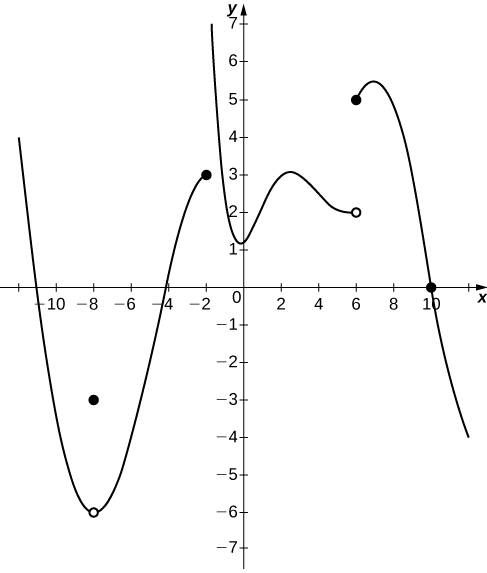13. $\underset{x\to 10}{\lim}f(x)=0$

14. $\underset{x\to 2}{\lim}f(x)=3$

15. $\underset{x\to -8}{\lim}f(x)=f(-8)$

16. $\underset{x\to 6}{\lim}f(x)=5$

In the following exercises, use the following graph of the function $y=f(x)$ to find the values, if possible. Estimate when necessary.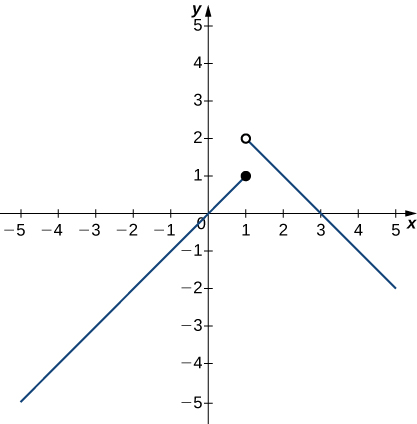17. $\underset{x\to 1}{\lim}f(x)$

18. $\underset{x\to 2}{\lim}f(x)$

19. $f(1)$

In the following exercises, use the graph of the function $y=f(x)$ shown here to find the values, if possible. Estimate when necessary.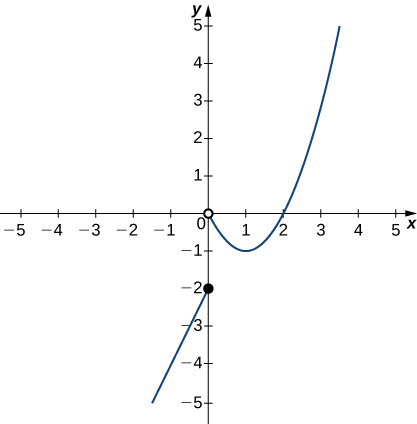20. $\underset{x\to 0}{\lim}f(x)$

21. $\underset{x\to 1}{\lim}f(x)$

22. $\underset{x\to 2}{\lim}f(x)$

23. $\underset{x\to 3}{\lim}f(x)$

In the following exercises, use the limit laws to evaluate each limit. Justify each step by indicating the appropriate limit law(s).

24. $\underset{x\to 0}{\lim}(4x^2-2x+3)$

25. $\underset{x\to 1}{\lim}\frac{x^3+3x^2+5}{4-7x}$

26. $\underset{x\to -2}{\lim}\sqrt{x^2-6x+3}$

27. $\underset{x\to -1}{\lim}(9x+1)^2$

In the following exercises, use direct substitution to evaluate each limit.

28. $\underset{x\to 7}{\lim}x^2$

29. $\underset{x\to -2}{\lim}(4x^2-1)$

30. $\underset{x\to 0}{\lim}\frac{1}{1+ \sin x}$

31. $\underset{x\to 2}{\lim}e^{2x-x^2}$

32. $\underset{x\to 1}{\lim}\frac{2-7x}{x+6}$

33. $\underset{x\to 3}{\lim}\ln e^{3x}$

In the following exercises, use direct substitution to show that each limit leads to the indeterminate form 0/0. Then, evaluate the limit.

34. $\underset{x\to 4}{\lim}\frac{x^2-16}{x-4}$

35. $\underset{x\to 2}{\lim}\frac{x-2}{x^2-2x}$

36. $\underset{x\to 6}{\lim}\frac{3x-18}{2x-12}$

37. $\underset{h\to 0}{\lim}\frac{(1+h)^2-1}{h}$

38. $\underset{t\to 9}{\lim}\frac{t-9}{\sqrt{t}-3}$

39. $\underset{h\to 0}{\lim}\frac{\frac{1}{a+h}-\frac{1}{a}}{h}$, where $a$ is a real-valued constant

40. $\underset{\theta \to \pi}{\lim}\frac{\sin \theta}{\tan \theta}$

41. $\underset{x\to 1}{\lim}\frac{x^3-1}{x^2-1}$

42. $\underset{x\to 1/2}{\lim}\frac{2x^2+3x-2}{2x-1}$

43. $\underset{x\to -3}{\lim}\frac{\sqrt{x+4}-1}{x+3}$

In the following exercises, assume that $\underset{x\to 6}{\lim}f(x)=4, \, \underset{x\to 6}{\lim}g(x)=9$, and $\underset{x\to 6}{\lim}h(x)=6$. Use these three facts and the limit laws to evaluate each limit.

44. $\underset{x\to 6}{\lim}2f(x)g(x)$

45. $\underset{x\to 6}{\lim}\frac{g(x)-1}{f(x)}$

46. $\underset{x\to 6}{\lim}(f(x)+\frac{1}{3}g(x))$

47. $\underset{x\to 6}{\lim}\frac{(h(x))^3}{2}$

48. $\underset{x\to 6}{\lim}\sqrt{g(x)-f(x)}$

49. $\underset{x\to 6}{\lim}x \cdot h(x)$

50. $\underset{x\to 6}{\lim}[(x+1)\cdot f(x)]$

In the following exercises, use the following graphs and the limit laws to evaluate each limit.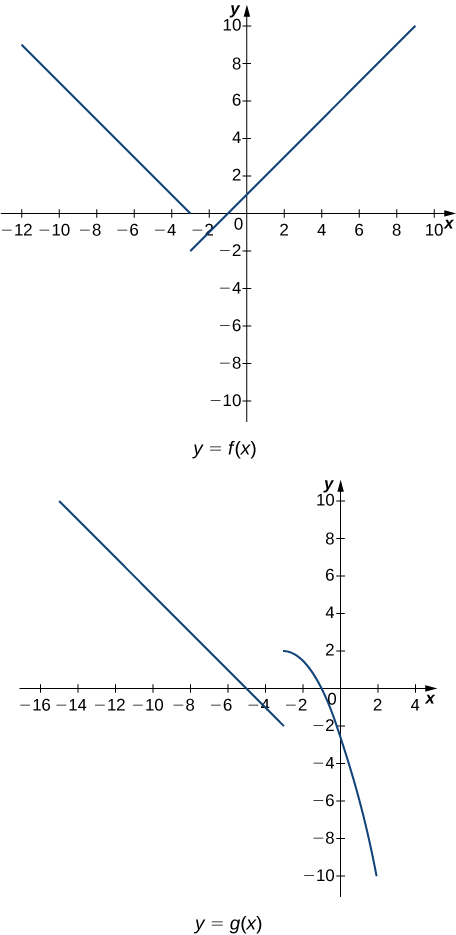51. $\underset{x\to 0}{\lim}\frac{f(x)g(x)}{3}$

52. $\underset{x\to -5}{\lim}\frac{2+g(x)}{f(x)}$

53. $\underset{x\to 1}{\lim}(f(x))^2$

54. $\underset{x\to 1}{\lim}\sqrt{f(x)-g(x)}$

55. $\underset{x\to -7}{\lim}(x \cdot g(x))$

56. $\underset{x\to -9}{\lim}[xf(x)+2g(x)]$

For the following problems, evaluate the limit using the Squeeze Theorem. Use a calculator to graph the functions $f(x), \, g(x)$, and $h(x)$ when possible.

57. [T] True or False? If $2x-1\le g(x)\le x^2-2x+3$, then $\underset{x\to 2}{\lim}g(x)=0$.

58. [T]$\underset{\theta \to 0}{\lim}\theta^2 \cos(\frac{1}{\theta})$

59. $\underset{x\to 0}{\lim}f(x)$, where $f(x)=\begin{cases} 0 & x \, \text{rational} \\ x^2 & x \, \text{irrational} \end{cases}$

## Glossary

infinite limit
A function has an infinite limit at a point $a$ if it either increases or decreases without bound as it approaches $a$
intuitive definition of the limit
If all values of the function $f(x)$ approach the real number $L$ as the values of $x(\ne a)$ approach $a$, $f(x)$ approaches $L$
one-sided limit
A one-sided limit of a function is a limit taken from either the left or the right
vertical asymptote
A function has a vertical asymptote at $x=a$ if the limit as $x$ approaches $a$ from the right or left is infinite